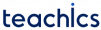# Sparse Matrix

A matrix is a two-dimensional array made of m rows and n columns, and so m x n elements. A matrix is called a sparse matrix if most of its elements are 0. An example of a sparse matrix of order 3 x 4 is shown below.

It is worth noting that matrix A of order 3 x 5 has 11 zero elements out of a total of 15. The usage of a 2D array to represent a sparse matrix wastes a lot of memory because the zeroes in the matrix are useless in most cases. This leads us to seek an alternate representation that could reduce the memory space consumed by zero entries.

A sparse matrix can be easily represented by a list of triplets of the form (Row, Column, Element). We store only the index of the row, index of column and value of the non zero elements.

### Triplet Representation of Sparse Matrix

The matrix A and its corresponding triplet representation is shown in the figure. The first row in the above table structure indicates the row number, the second row represents the column number, and the third column represents the non-zero value at index [row, column]. The size of the table changes with the number of non-zero elements in the sparse matrix.

From the table representation, we can identify the non zero elements in the sparse matrix. Value 5 is available in the 0th row and 0th column. In the 0th row and 1st column, value 3 is stored. Similarly, value 1 and 2 is stored at index [1,3] and [2,2] respectively.

### Example: Array Implementation of Sparse Matrix in C

```// C program for Sparse Matrix Representation
// using Triplets
#include<stdio.h>
int main()
{
int S,m,n,i,k=0,size=0;

//size of matrix
printf("Enter number of rows in the matrix : ");
scanf("%d",&m);
printf("Enter number of columns in the matrix : ");
scanf("%d",&n);

printf("Enter elements in the matrix : ");
for (int i = 0; i < m; i++)
for (int j = 0; j < n; j++)
scanf("%d",&S[i][j]);

//print original matrix
//find number of non-zero elements
printf("The matrix is \n");
for (int i = 0; i < m; i++) {
for (int j = 0; j < n; j++) {
printf(" %d ",S[i][j]);
if (S[i][j] != 0)
size++;
}
printf("\n");
}

//new matrix fr triplet representation
int M[size];

//making of new matrix
for (int i = 0; i < m; i++)
for (int j = 0; j < n; j++)
if (S[i][j] != 0)
{
M[k] = i;
M[k] = j;
M[k] = S[i][j];
k++;
}

//print new matrix
printf("Triplet representation of the matrix is \n");
for (int i=0; i<3; i++)
{
for (int j=0; j<size; j++)
printf(" %d ", M[i][j]);

printf("\n");
}
return 0;
} ```

#### Output

```Enter number of rows in the matrix : 3
Enter number of columns in the matrix : 5
Enter elements in the matrix : 5 3 0 0 0
0 0 0 1 0
0 0 2 0 0
The matrix is
5  3  0  0  0
0  0  0  1  0
0  0  2  0  0
Triplet representation of the matrix is
0  0  1  2
0  1  3  2
5  3  1  2```
Subscribe
Notify of